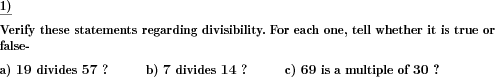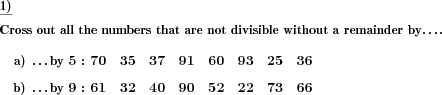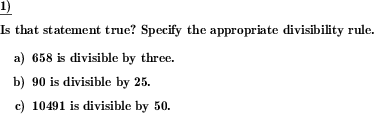Custom math worksheets at your fingertips# Details for problem "Factor or multiple?"

Quickname: 7651

Elementary School, Primary School, Junior High School, Middle School.

## Summary

Determine whether a number is a factor or multiple of another.

## Example## Description

For two whole positive numbers n and m, it has to be determined whether the statements

• n divides m
• n does not divide m
• n is a multiple of m
• n is not a multiple of m

are true.

The number of problems can be chosen, and the number range is selectable. It is possible to specify what is asked for: "divides", "is a multiple of" or a random mix of both.

For the notation of "divides", there is an option to choose between the notation of "a divides b" or "a | b".

Download free printable worksheets for this math problem here. The worksheet contains the problems only, the solution sheet includes the answers. Just click on the respective link.

•Worksheet 1Solution sheet with answers
•Worksheet 2Solution sheet with answers
•Worksheet 3Solution sheet with answers

If you can not see the solution sheets for download, they may be filtered out by an ad blocker that you may have installed. If this is the case, please allow ads for this page and reload the page. The solution sheets will then reappear.

• Do these sample worksheets do not really fit?
• Do you need more math worksheets, with a different level of difficulty?
• Would you like to combine different problems on a worksheet and adjust them to your needs?
• As a teacher, you can put together your own worksheets using the automatically generated math problems provided.
With a free initial credit, you can start creating your own math worksheets in a few minutes.

You can try it for free! Register here, to create custom worksheets now!

## Customization options for this problem

Parameter
Possible values
Number of problems
1, 2, 3, 4, 5, 6, 7, 8, 9, 10
Number range
10, 20, 30, 40, 50, 100
mixed, factor, multiple
Notation for "divides"
3 | 12, 3 divides 12

## Similar problems

Remark
Description
Find gcd and lcm of two numbers
For two given numbers, the gcd and lcm are determined by comparing lists of multiples or divisors
Also refer to divisibility rules
The rules on divisibility have to be specified and applied.

## Other types of problems that appear on worksheets with this problem:

Relevance
Name
Description
Quickname
Example
****
Test for divisibility
A row of numbers is presented. Delete those that are not divisible by a given divisor.****
Divisibility rules to be specified and applied
The rules on divisibility have to be specified and applied.Deutsche Version dieser Aufgabe
These informational pages with samples describe math problems that can be combined on custom math worksheets with solutions for home and K-12 school use.
Deutsche Seiten
×